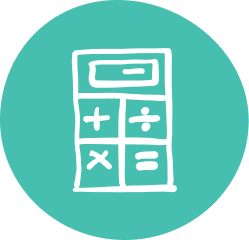### Quality Grade 9 Math Tutoring & Help

Our 9th Grade math tutoring gives teens the skills necessary to carry on studying math while igniting a passion for learning.

Oxford Learning®’s Grade 9 math tutoring program is designed to help students develop thinking and learning skills to help make understanding math simpler. Grade 9 math tutors not only make understanding math concepts easier, but also help students think actively and approach math with more confidence.

At Oxford Learning®, we believe that learning is about more than better grades. Our cognitive approach helps teens learn how to learn, helping make learning carry on even after the textbook is closed.

Here at Oxford Learning® our Grade 9 math help program teaches students to excel in both the academic and the applied math courses.

### Grade 9 Math Tutoring Subjects

##### Academic: Number Sense & Algebra
• Manipulating expressions and solving equations
• Operating exponents
• Linear relations
• Investigate relationships using data management
• Connect various representations of linear relations
• Relationship between the relation of an equation and its graph
• The properties of a slope
• Use the properties of linear relations to solve problems
• Investigate the optimal values of measurements
• Solve problems involving perimeter, area, surface area and volume
• Investigate and apply geometric relationships
##### Applied: Number Sense & Algebra
• Solve problems involving proportional reasoning
• Simplifying expressions
• Solving equations
##### Applied: Linear Relations
• Using data management to investigate relationships
• Determining the characteristics of linear relations
• Investigating the constant rate of change
• Connecting different representations of linear relations and solving problems using the representations
##### Applied: Measurement & Geometry
• The optimal values of the measurements of rectangles
• How to solve problems using perimeter, area and volume
• Investigating and applying geometric relationships